# Length of a Circle: Iterative Numerical Approximation

The equation of a general circle centered at the point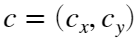with radious r is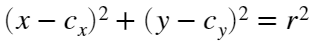it is known that its length is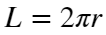. We want to approach the actual length by an increasing value of the inscrived polygon length.

## Iterative approach

Let's illustrate the idea by the following plot: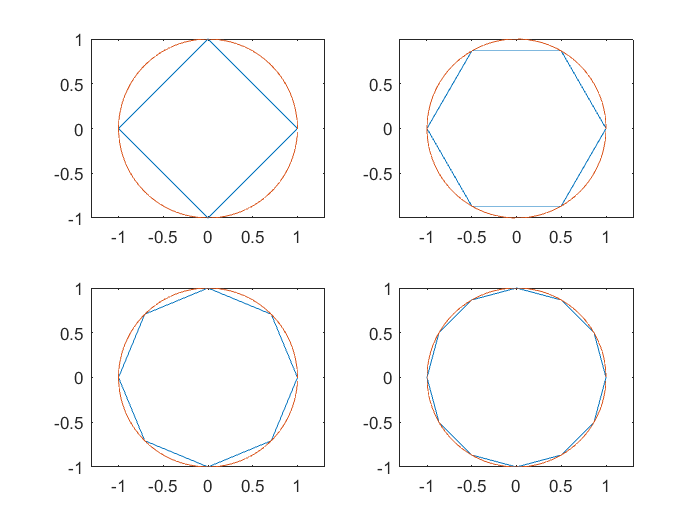## Generate the points of the inscribed polygon

We'll use polar coordinates to generate the points on the cercle: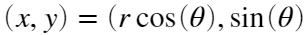where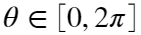r = 1;
numPoints = 10;
ang=0;
pasAng=2*pi/numPoints;
for i = 1:numPoints
x(i) = r*cos(ang);
y(i) = r*sin(ang);
ang=ang+pasAng;
end
points=[x',y'];
points=[points;x(1),y(1)]; %to close the polygon
% Plot the inscribed polygon
angC=0:0.05:2*pi; %take more points to draw the circle
xx=cos(angC);
yy=sin(angC);
xx=[xx,xx(1)]; % to close the cercle
yy=[yy,yy(1)]; % to close the cercle
plot(xx,yy,'b'); %plot the the circle
hold on;
plot(points(:,1), points(:,2),'r'); %plot the polygon
hold off;
axis equal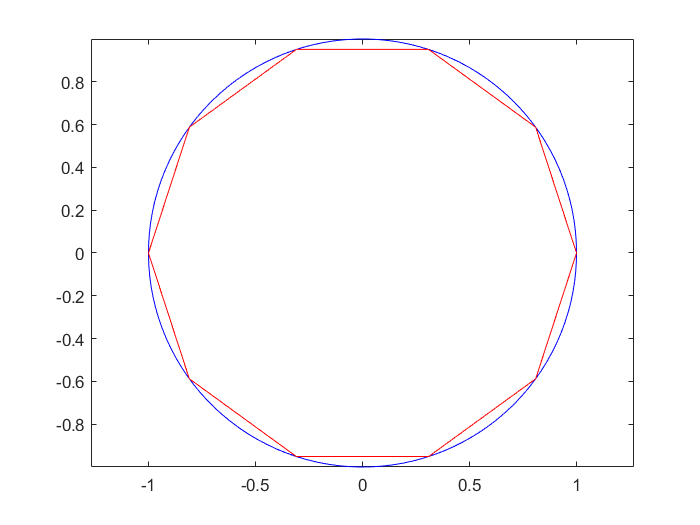Now we compute the length of the closed curve associated to this polygon (the perimeter).
Each edge between points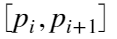has length: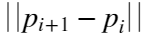perimeter = 0;
for i = 1:numPoints
perimeter = perimeter+norm(points(i+1,:)-points(i,:));
end

## Check the error

Finally the error obtained by the present approximation is:
actualLength = 2*pi*r;
absError = abs(perimeter-actualLength);
relError = absError/actualLength;
fprintf('nPoints= %d, perimeter = %e, absErr= %e, relErr= %e \n',numPoints,perimeter,absError,relError);
nPoints= 10, perimeter = 6.180340e+00, absErr= 1.028454e-01, relErr= 1.636836e-02

## Exercise 1:

Build a function returning the points coordinates vector of the inscribed polygon given the radious and the number of points
function points = generatePolygonPoints(r,numPoints)

## Exercise 2:

Change the number of points and see how the length is better approximate.

## Exercise 3: Approximate the length of a circle up to a desired precission.

The question now is to compute how many points are needed to approximate the
actual length of the circle with the perimeter of the inscrived polygon. For that, we will use a modification of the previous script, following the next steps:
Step 1: Define a tolerance precission variable, tol = 1.e-5
Initialize the numPoints variable to 8.
Step 2: Use a while loop until the absError is less than the tolerance
while(absError > tol)
generate the points
compute the present Length
compute the present absError
increment numPoints
end
Step 3:
Show in the commad window the computed values for the numPoints variable
Solution= For radious r = 10 and tol = 1.e-5, numPoints= 3215 are needed